## Figures index

#### Abdulrahman Almutairi, Pericles Pilidis, Nawaf Al-Mutawa

American Journal of Energy Research. 2016, 4(1), 1-10 doi:10.12691/ajer-4-1-1• Figure 1. Schematic diagram of the SCGT and RHGT systems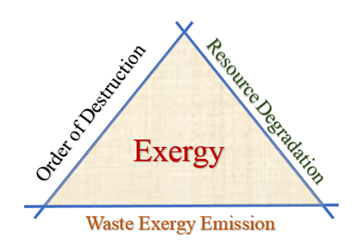• Figure 2. Exergy analysis of three forms of environmental damage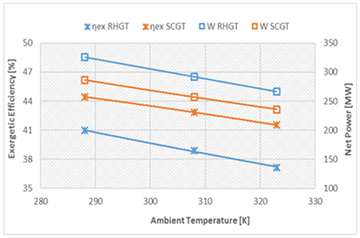• Figure 3 Relationship between ambient temperature, exergetic efficiency and net power output• Figure 4. Exergetic efficiency versus load variations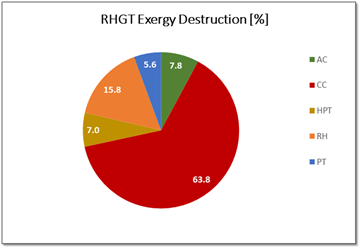• Figure 5. Exergy destruction for RHGT system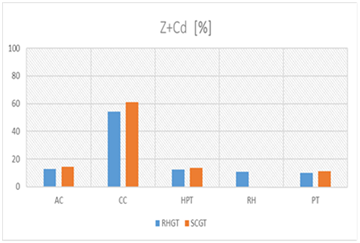• Figure 6. The relative percentages of exergy destruction and component cost ( ) for RHGT and SCGT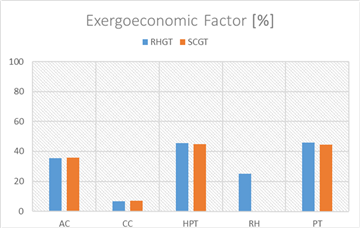• Figure 7. Exergoeconomic factors for engine components for both RHGT and SCGT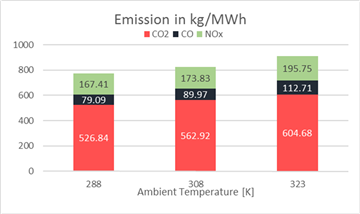• Figure 8. The CO2 and pollutant emissions for RHGT per MWh of electrical energy generated for three ambient temperatures• Figure 9. The CO2 and pollutant emissions for SCGT per MWh of electrical energy generated for three ambient temperatures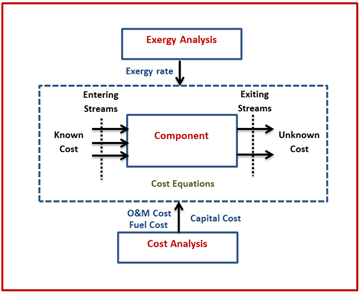• Figure A 1. Block Diagram of SPECO Method